### 2.2.2 Doping, Carrier Density, Mobility, and ConductivityAgain, we look at a "perfect" semiconductor, where doping has been achieved by replacing some lattice atoms by suitable doping atoms without – in the ideal world – changing anything else.We have now additional allowed energy levels in the band gap, belonging to the doping atoms, i.e. they are localized states.These levels may or may not be occupied by electrons; the Fermi distribution will give the probability for occupancy as before. In analogy to the intrinsic case, we now have the following highly stylized picture: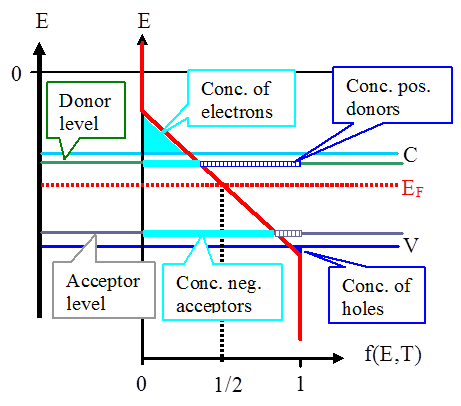As in the intrinsic case, in this drawing no distinction was made between carrier density (in cm–3) and carrier concentration (in ppm, ppb, or the like).

Charge Neutrality ConditionWhile the picture may look complicated, it is actually just a means to keep track of the number of charges in the semiconductor. Since there is an over-all charge neutrality, we have
 S pos. charges = S neg. chargesThis equation can be used to calculate the exact position of the Fermi energy (as we will see). Since almost everything else follows from the Fermi energy, let's look at this in some detail.But wait – why are there positive charges at all? Aren't we just discussing the occupation of electronic levels/states? After all, electrons are negatively charged. And by which rule is it that some states in the above figure count the one way (negative), but some the other way (positive)?This rule is simple: Everything counts relative to the individual behavior at zero kelvin (*) where the conduction band is completely empty, the valence band is completely filled, all donor states are occupied, and all acceptor states are empty.Of course, if both donor and acceptor states were present together in a material, then at zero kelvin the electrons would not be distributed like that. And that's why it is emphasized that one has to look at the individual behavior of these states at zero kelvin, because charge neutrality is defined relative to the latter (for which it obviously holds).(* Yes: The units of measurement are spelled with small letters – because it's only the natural persons' names that are spelled with capital letters.)First, let's count the negative charges which, following the above rule, means to look for those places where at zero kelvin no electrons are to be found.There are, first, the electrons in the conduction band. Their density, as spelled out before, was
ne = Neffe –  · exp æ ç è ö ÷ øMore generally and more correctly, however, we have
ne  = Neffe · f(EC, EF ,T)The Fermi energy EF is now included as a variable in the Fermi function, because the density of electrons depends on its precise value which we do not yet know. In this formulation the electron density comes out always correct, no matter where the Fermi energy is positioned in the band gap.Then there are, second, the negatively charged acceptor atoms . Their density NA is given by the density of acceptor states (which is just the doping density N A ) times the probability that the states are occupied, and that is given by the value of the Fermi distribution at the energy of the acceptor state, f(EA, EF,T). We have accordingly
NA  =  NA · f(EA, EF,T)Note: The Fermi distribution in this case should be slightly modified to be totally correct. The difference to the straight-forward formulation chosen here is slight, however, so we will keep it in this simple way. More about thisin an advanced module.Now let's count the positive charges which, following the above rule, means to look for those places that at zero kelvin are completely filled with electrons.First , we have the holes in the valence band. Their number is given by the number of electrons that do not occupy states in the valence band; in other words we have to multiply the effective density of states with the probability that the state is not occupied.The probability that a state is not occupied is just 1 minus the probability that it is occupied, or simply 1 – f(E, EF, T). This gives the density of holes to
 nh  =  Neff 1  –  f(EV h · , EF , T) æ è ö øSecond , we have the positively charged donors, i.e. the donor atoms that lost an electron. Their density ND+ is equal to the density of states at the donor level (which is again identical to the density of the donors themselves) times the probability that the level is not occupied; so, we have
ND+  = æ è ö øCharge neutrality thus demands
 Neffe 1 – f(EV, EF,  +  ND · 1 – f(ED, · f(EC , EF, T)  +  NA· T) EF, T) f (EA, EF, T)  =  Neff h  · æ è ö ø æ è ö øIf we insert the expression for the Fermi distribution
f( En, EF, T)  =

1
exp æ
è
En  –  EF
kT
ö
ø
+ 1where En stands for EC,V,D,A , we have, for a given material with a given doping, one equation for the one unknown quantity EF !Solving this equation for any given semiconductor and any density of (ideal) donors and acceptors will not only give us the exact value of the Fermi energy EF for any temperature T, i.e. EF (T), but all the carrier densities as specified above.Unfortunately, this is a messy transcendental equation – it has no direct solution that we can write down.Before the advent of cheap computers, this was a problem – you had to do case studies and use approximations: High or low temperatures, only donors, only acceptors and so on. This is still very useful, because it helps to understand the essentials.However, here we could use a program (written by J. Carstensen) that solves the transcendental equation and provides all functions and numbers required.Unfortunately, the relevant JAVA applet doesn't work anymore, so the link to this illustration module is deactivated.Therefore, here we show only a screen shot with the result for the typical case of Si with1. An acceptor density of 1015 cm–3 (red line).2. A donor density of 1017 cm–3 (blue line).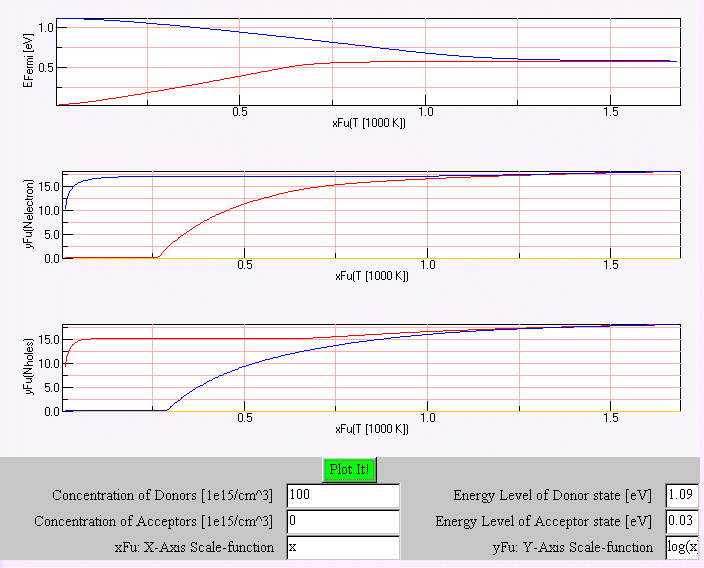One of many important points to note about carrier densities is the simple, but technologically supremely important fact that the majority carrier density for many semiconductors in a technically useful temperature interval is practically identical to the dopant density.This is then the temperature regime were we can hope to have only a weak temperature dependence of electronic properties and thus of devices made from Si.The equations used for charge neutrality also make possible to deduce an extremely important relation, the mass action law (for electrons and holes), as follows:First we consider the product
ne · nh  =  N effe · f(EC, EF, T) · Neffh · [1 – f(EV, E F,T)]Then we insert the formula for the Fermi distribution and note that
1 – f(EV, T)  =  1   –

1
=

1
exp æ
è
EV  –  EF
kT
ö
ø
+  1 1  +  exp æ
è
EF  –  EV
kT
ö
øUsing the Boltzmann approximation for the Fermi distribution, we get the famous and very important mass action law (try it yourself!):
 ne – · nh  =  Neffe · Neffh ·  exp æ ç è ö ÷ øWe are now in a position to calculate the density of majority and minority carriers with very good precision if we use the complete formula, and with a sufficient precision for the appropriate temperature range where we can use the following very simple relations:
nmaj  =  Ndop

n min  =  n i2
nmaj
=  ni2
NdopWe know that the conductivity s of the semiconductor is given by
s =  e · (n e · µe  +  nh· µh)With µ = mobility of the carriers.We now have the densities; obviously we now have to consider the mobility of the carriers.

MobilityFinding simple relations for the mobility of the carriers is just not possible. Calculating mobilities from basic material properties is a far-fetched task, much more complicated and involved than the carrier density business.However, the carrier densities (and their redistribution in contacts and electrical fields) is far more important for a basic understanding of semiconductors and devices than the carrier mobility.At this point we will therefore only give a cursory view of the essentials relating to the mobility of carriers.The mobility µ of a carrier in an operational sense is defined as the proportionality constant between the average drift velocity vD of an ensemble of carriers in the presence of an electrical field E:
vD  =  µ · EThe average (absolute) velocity v of a carrier and its drift velocity vD must not be confused; for a detailed discussion consult the links to two basic modules:The simple linear relationship between the drift velocity and the electrical field as a driving force is pretty universal – it is the requirement for ohmic behavior (look it up in the link) – but not always obeyed. In particular, the drift velocity may saturate at high field strengths, i.e. increasing the field strength does not increase vD anymore. We come back to that later.Here we only want to get a feeling for the order of magnitudes of the mobilities and the major factors determining these numbers.As we (should) know, the prime factor influencing mobility is the average time between scattering processes. In fact, the mobility µ may be written as
µ  =  e · t s
mWith ts = mean scattering time. We thus have to look at the major scattering processes in semiconductors.There are three important mechanisms:The first (and least important one) is scattering at crystal defects like dislocations or (unwanted) impurity atoms.Since we consider only "perfect" semiconductors at this point, and since most economically important semiconductors are pretty perfect in this respect, we do not have to look into this mechanism here.However, we have to keep an open mind because semiconductors with a high density of lattice defects are coming into their own (e.g. GaN or CuInSe2 ) and we should be aware that the mobilities in these semiconductors might be impaired by these defects.Second, we have the scattering at wanted impurity atoms, in other word at the (charged) dopant atoms.This is a major scattering process which leads to decreasing mobilities with increasing doping density. The relation, however, is non-linear and the influence is most pronounced for larger doping densities, say beyond 1017 cm–3 for Si.Examples for the relation between doping and mobilities can be found in the illustration.As a rule of thumb for Si, increasing the doping level by 3 orders of magnitude starting at about about 1015 cm–3 will decrease the mobility by one order of magnitude, so the change in conductivity will be about only two orders of magnitude instead of three if only the carrier density would change.The scattering at charged dopant atoms decreases with increasing temperature. (This results mainly from the scattering being Rutherford scattering via Coulomb interaction, for which the scattering cross section reduces with increasing temperature.)Third, we have scattering at phonons – the other important process. Phonons are the (quantum-mechanical) "particles" corresponding to the thermally stimulated lattice vibrations and thus strongly depend on temperature.This part scales with the density of phonons, i.e. it increases with increasing temperature (with about T 3/2). It is thus not surprising that it dominates at high temperatures (while scattering at dopant atoms may dominate at low temperatures).Scattering at phonons and dopant atoms together essentially dominate the mobilities.The different and opposing temperature dependencies almost cancel each other to a certain extent for medium to high doping levels (see the illustration), again a very beneficial feature for technical applications where one doesn't want strongly temperature dependent device properties.

 ConductivityWith a relatively simple law for the carrier densities and no direct equations for the mobility, but relatively simple behavior of the mobility, conductivity data can now be compiled.Some data giving the relationship between doping and resisitivity (=1/conductivity) or the temperature dependence of the conductivity are shown in the illustration.The illustrations show different behavior for n- and p-type Si, which could not be predicted in our present simplified treatment; it is due to different mobilities of the different carriers.Our present assumptions of equal parameters (mass, mobility, life time, and so on) for electrons and holes, while justifiable in a 1st degree approximation, are too simple. Specific differences between holes and electrons exist and will be dealt with in subsequent chapters. They are responsible for the differences in mobility, conductivity, and so on between holes and electrons.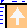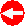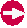© H. Föll (Semiconductors - Script)